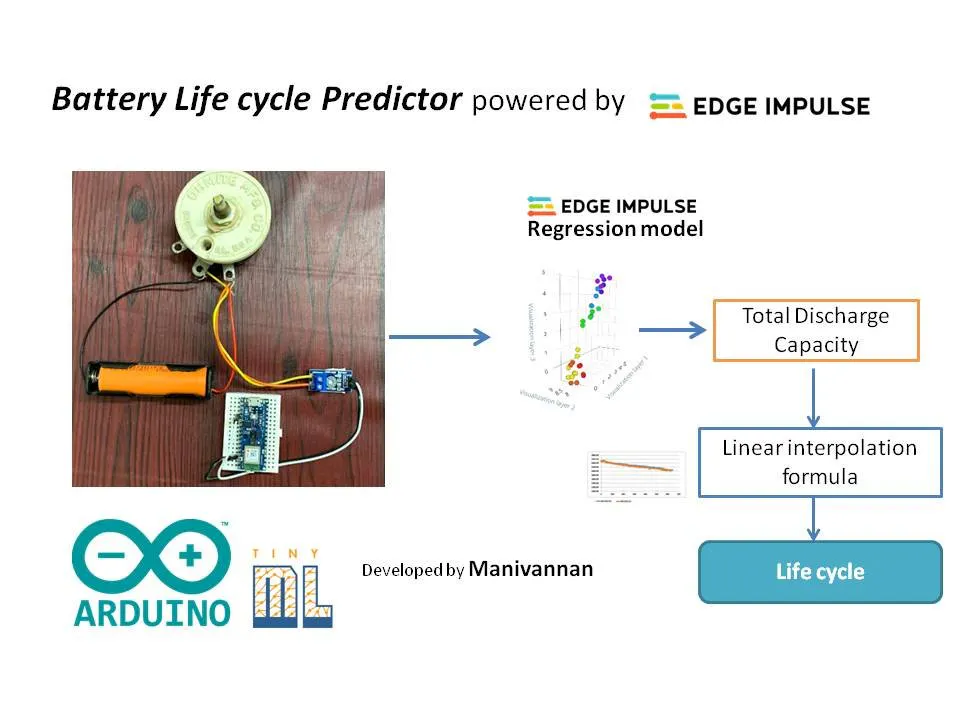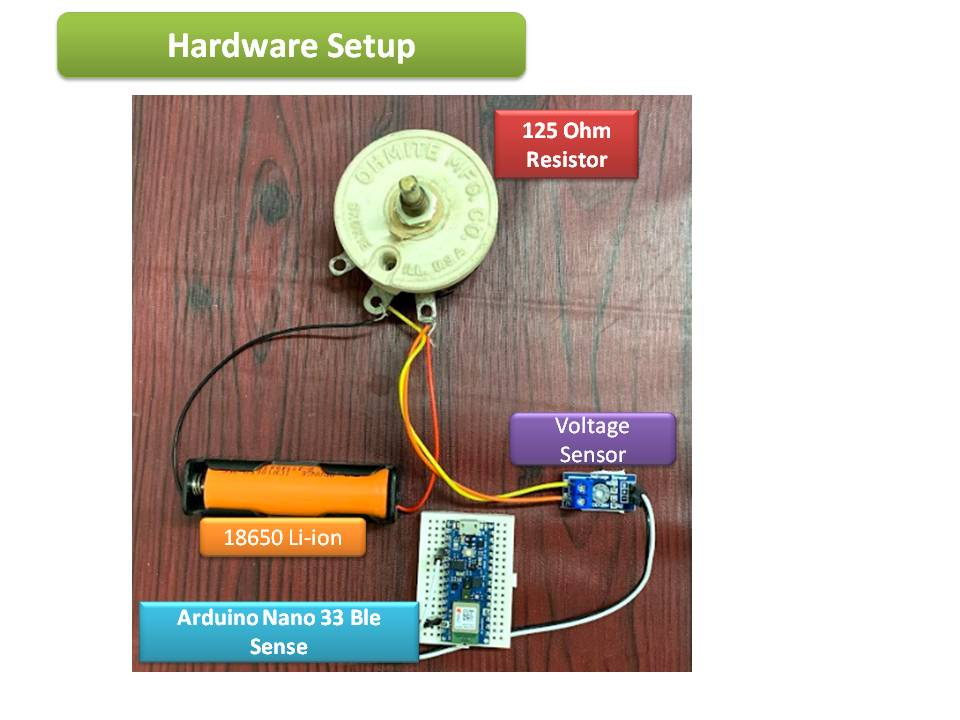Project tutorialA TinyML model to predict the Lithium Ion battery's life cycle within shorter time using Edge Impulse.

• 1,994 views
• 17 respects

## Apps and online services

Before the detailed documentation, I have attached the demovideo of my project in below YouTube link, Kindly watch it.

To predict the Life cycle of Lithium Ion battery, there are many methods are available. One of the common method is by linear interpolating the discharge capacity to the life cycle.

In a conventional method, To estimate the life cycle using discharge capacity method, it takes 2 hours 30 mins of time for 2500mAh - single Lithium Ion cell.

### Technology contribution:

I developed a TinyML model using Edge impulse based on Linear regression method to predict the life cycle of a battery in 1 hour time period.

This could save 90 mins of time compared to normal conventional method.

### Know About Lithium Ion battery 18650 Type:

Before proceeding with technical work, let's go through the basic specification about Lithium Ion battery.

• The nominal voltage is 3.7v which is the actual voltage of fully charged battery when it is connected to Load.
• The capacity of battery is 2500mAh, So If the battery is discharging at constant current 1A, then it takes 1 hour to discharge 1000mAh and for 2500mAh, it takes 2 hours and 30 mins.
• When the battery discharge voltage reaches 2.75 v, it can be considered as fully discharged.

### Architecture:

The Battery Life cycle predictor involves :

2. Simulating different discharge voltage patterns

3. Training the Model

4. Deployment

5. Applications

To read the discharge battery voltage, the voltage sensor is connect parallel to the rheostat. The Rheostat is adjusted in order to set the discharge current as 1 Amps.

Once the rheostat is connected to consume 1A load current, then the voltage reading had been displayed in serial terminal with 1 minute sample time.

### Hardware Setup:

``// Define analog input#define ANALOG_IN_PIN A0 // Floats for ADC voltage & Input voltagefloat adc_voltage = 0.0;float in_voltage = 0.0; float cal_temp=0.33;// Floats for resistor values in divider (in ohms)float R1 = 30000.0;float R2 = 7500.0;  // Float for Reference Voltagefloat ref_voltage = 5.0; // Integer for ADC valueint adc_value = 0;int minute=0;  void setup(){   // Setup Serial Monitor   Serial.begin(115200);} void loop(){   // Read the Analog Input   adc_value = analogRead(ANALOG_IN_PIN);      // Determine voltage at ADC input   adc_voltage  = (adc_value * ref_voltage) / 1024.0;       // Calculate voltage at divider input   in_voltage = adc_voltage / (R2/(R1+R2));       // Print results to Serial Monitor to 2 decimal places  //Serial.print("Input Voltage = ");  Serial.print(minute);  Serial.print(',');  Serial.println(in_voltage-cal_temp, 2);  minute++;    // Short delay  delay(60000);}``

### 2. Simulating different discharge voltage patterns

2.1 Save the normal battery discharge data in excel

copy and save the serial monitor data into the excel file as below template. The voltage value should be in second column under 'Y'. The first column is time series. it should be incremented as ( 1) 1000ms.

Step2.2 : Signal builder in Matlab

Create a new Simulink model in Matlab

Then type 'signal builder' in workspace and select it. Also insert

the 'scope' to connect it to the signal builder. Please refer the below screenshot.

Load the battery voltage data in signal builder and edit it according to different life cycle patterns.

Then export the file and copy and save it as csv file.

### 3. Training the model using Edge Impulse

Once the datasets for different voltage are generated, then upload the csv file in Edge Impulse.

since we are going to train in regression model, The label of a dataset should be numeric one.

In our case, the battery voltage after one hour as a label.

3.1 Create Impulse

In create impulse section, mention 28000ms as window time.

[ 28 minutes data is manipulated as 28 sec data, since we had taken a data in one minute sample time]

Since it is a raw data and regression model, no DSP block is required.

In a generating features, the expected voltage from 2.75 to 2.90 is listed.

2.75v at 2 hour is 2000mAh capacity, where as 2.9v at 2 hour time period is 2500mAh. The linear interpolation can calculate the estimated capacity by predicting the voltage.

Train the model with below settings.

### Model Testing

I have tested with two datasets where the 100% accuracy is achieved.

### Deployment:

Deploy it as Arduino library.

And include the library in Arduino as library file.

### Application Code:

The battery life cycle is predicted by linear interpolating Discharge capacity to the life cycle.

In EI model, the model will predict the estimated battery voltage level after 1 hour. ( at 120th minute). The predicted voltage will be linear interpolated to the discharge capacity as below:

X axis : xo = 2.9, x1=2.75

Y axis : yo= 2500, y1=2000

The predicted discharge capacity can be used to estimate the life cycle.

From specification of 18650 Li ion battery type, the life cycle of battery for discharge capacity is plotted. This will be again linear interpolated to estimate the battery Life cycle.

### Application

The reuse of Lithium Ion battery will have huge market demand in future. So this kind of ML model to save the time in predicting the life cycle will create a huge impact in industries.

## Code

##### Battery Life cycle detector
Unzip the file in github and include the library in arduino . The run the application arduino code

## Schematics

connect as per diagram#### Similar projects you might like

Project tutorial by Manivannan

• 15,794 views
• 70 respects

#### TinyML Water Sensor - Based on Edge Impulse & Arduino Sense

Project tutorial by Enzo

• 2,716 views
• 1 comment
• 13 respects

Project tutorial by Ish Ot Jr.

• 5,545 views
• 33 respects

#### Robot Fall Detection with Edge Impulse

Project tutorial by Arduino “having11” Guy

• 3,168 views
• 1 comment
• 9 respects

#### BeeMonitor

Project tutorial by Team 🐬 Delfinčki 🐬

• 10,145 views